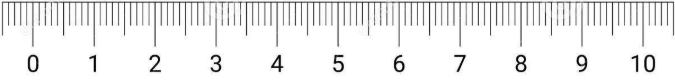# Dimensions in Computer Graphics

## Introduction

The image dimension in computer graphics refers to the width and length of a digital image. It is usually measured in terms of pixels, though it can also be measured in centimeters and inches in some graphics programs.

Before we learn about dimensions, let's turn away for a while from the digital world and learn something in our physical world.

We live in a 3-dimensional world and this means you can easily describe positions from everything, even tiny objects like sand in three dimensions. So you might wonder, how can 1 or 2-dimensional objects exist in a 3-dimensional universe? We can understand this well by comparing the global positioning system that is used to know the positions of the world and 3D CAD positioning in 3D modeling software.

A CAD program like SelfCAD uses XYZ (Cartesian coordinate) to provide details of the location of a 3D object. GPS on the other hand uses a (Spherical coordinate) longitude, latitude, and elevation as a system for 3D positioning. It’s worth noting that every object in both the physical universe and virtual 3D CAD software scene has a 3-dimensional position. And someone may ask, what makes a 3D object one dimensional, or two dimensional, or 3d- dimensional?

Obviously it is not related to positioning, so what is it? In order to explain this, we first need to learn about measurements. Volume and size are the two basic ways used for measuring a 3d object.

## How to measure the object's volume?

One of the old, sophisticated, and yet clever methods of measuring objects that are irregular, is usually based on water displacement. You simply submerge the object underwater and measure the height of the water level that has risen.

But suppose you submerge like a stone in a tube that has water, measuring 20ML, and the water level increases to 40 mL. This shows that the stone has a volume of 20 mL.

But you may wonder, what if there is a cavity in the object or if the object is hollow? You will get to know that objects that are hollow are measured by the use of wall thickness, but at the same time closed object measured by its volume. When one understands this, it becomes easier to understand the topology structure of an object.

## How to measure the size of the object?

To measure the size of an object, one needs to first measure the absolute distance between two points. It doesn’t matter which point one starts from or the direction one will go, you only count the units between the points, as shown below.Having looked at how you can measure the volume and size of an object, you may wonder, how can you measure the size of an object that has only one point of reference? This is where now the issue of dimensions comes into question.

## What is a one-dimensional object?

A dimensional object is an object in which it’s not possible to measure the size or distance because of the presence of one point of reference only. Example of 1D objects is one vertex, one pixel, a grain of salt.

## Which measuring Units do we use?

Everything can simply be measured in 3D, but one has to use a unit of measurement that is smaller. So technically, one dimensional and two-dimensional objects are usually classified on a relative basis. That is, according to a specific unit of one’s choice.

## What makes a two-dimensional object?

A two-dimensional object is created when there are two vertices involved. This means that the object distance and size can be measured hence it’s said to be a two-dimensional object.

If a second connected like is added to a single line it will form a parallel line, or it will be perpendicular to each other regardless of the direction. This will still be a two-dimensional object as one can be able to measure its size and distance. The same applies if more lines are added as long as a closed loop is not formed in the process.

## Creating an Area?

When there are at least three lines, a closed (triangle) loop will already be created and if another line is added it can form a rectangle. As soon as a closed shape is created, getting the area becomes easier.

For example, if one has a rectangle measuring 100 by 100 pixels rectangle, if the outer perimeter is measured, you get 400 pixels in size. But if the area is measured, you get 10,000. This is because the inner area can be filled with up to 10000 pixels.

When any unit is used, the same result will be formed as it is an absolute measurement.

## Area vs Volume

Based on the description above, the area is similar to volume in some sense, though the only difference is that an area is two dimensional hence can’t hold anything in its boundaries.

## What makes an object three dimensional?

An object is said to be a three-dimensional model if it has three dimensions, that is, length, height, and width. The difference between a two-dimensional model and a three-dimensional model is that a three-dimensional object has depth and thickness.

Other attributes found in three-dimensional objects are the presence of faces, vertices, and edges. Examples of basic three-dimensional objects are rectangular prism, cube, cylinder, and sphere.

## Can we get to higher dimensions?

It’s possible to go to a higher dimension in theory, though it hasn’t been known exactly what makes an object to be four-dimensional. This is because even if one keeps adding planes and angles on the object in various directions, it still ends up being a 3D dimensional.

Do you want to learn 3D modeling? Check out our interactive tutorials.